# Reproducing Polynomials with B-Splines

(Unterschied) ← Nächstältere Version | Aktuelle Version (Unterschied) | Nächstjüngere Version → (Unterschied)

A B-Spline of order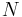$N$ is known to be able to reproduce any polynomial up to order$N$:$\sum_{n \in \mathbb{Z}} c_{m,n} \beta_N (t - n) = t^m$

In words, a proper linear combination of shifted versions of a B-Spline can reproduce any polynomial up to order$N$. This is needed for different applications, for example, for the Sampling at Finite Rate of Innovation (FRI) framework. In this case any kernel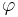$\varphi$ reproducing polynomials (that is, satisfying the Strang-Fix conditions) can be used. However, among all possible kernels, the B-Splines have the smallest possible support.

An important question is how to obtain the coefficients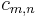$c_{m,n}$ for the reproduction-formula. In this small article, I describe one way.

Starting from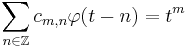$\sum_{n \in \mathbb{Z}} c_{m,n} \varphi(t - n) = t^m$

the coefficients can be obtained using the dual of$\varphi$,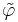$\tilde{\varphi}$ (I set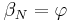$\beta_N = \varphi$ for consistency with my notes):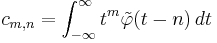$c_{m,n} = \int_{-\infty}^{\infty} t^m \tilde{\varphi}(t - n)\,dt$

However, even if the dual would be known, solving the infinite integral is only feasible when the dual has finite support. This is the case with the B-Spline itself but not with its dual!

A closer look at the formula tells that this is nothing more than a convolution (under the assumption that$\tilde{\varphi}$ is symmetric which is the case):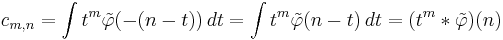$c_{m,n} = \int t^m \tilde{\varphi}(-(n-t))\,dt = \int t^m \tilde{\varphi}(n-t)\,dt = (t^m * \tilde{\varphi})(n)$

Now, this can be transformed to fourier domain: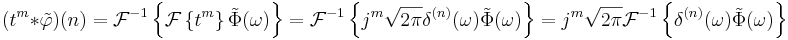$(t^m * \tilde{\varphi})(n) = \mathcal{F}^{-1}\left\{ \mathcal{F}\left\{t^m\right\} \tilde{\Phi}(\omega)\right\} = \mathcal{F}^{-1}\left\{ j^m \sqrt{2\pi} \delta^{(n)}(\omega) \tilde{\Phi}(\omega) \right\} = j^m \sqrt{2\pi} \mathcal{F}^{-1}\left\{\delta^{(n)}(\omega) \tilde{\Phi}(\omega) \right\}$

Writing the inverse of this expression yields: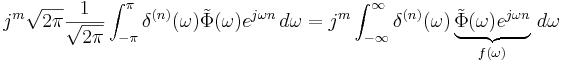$j^m \sqrt{2\pi} \frac{1}{\sqrt{2\pi}} \int_{-\pi}^{\pi} \delta^{(n)}(\omega) \tilde{\Phi}(\omega) e^{j\omega n}\,d\omega = j^m \int_{-\infty}^{\infty} \delta^{(n)}(\omega) \underbrace{\tilde{\Phi}(\omega) e^{j\omega n}}_{f(\omega)}\,d\omega$

It is known that: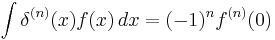$\int \delta^{(n)}(x) f(x)\,dx = (-1)^n f^{(n)}(0)$

so that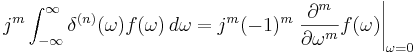$j^m \int_{-\infty}^{\infty} \delta^{(n)}(\omega) f(\omega)\,d\omega = j^m (-1)^m \left. \frac{\partial^m}{\partial \omega^m} f(\omega) \right|_{\omega = 0}$

Now the whole procedure has been reduced to calculating the derivative of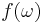$f(\omega)$ and set the result to zero.

An open question is how to obtain the dual of$\varphi$. As the reproduction formula spans a vector space,$\varphi$ must be at least bi-orthogonal to$\tilde{\varphi}$. This translates in fourier domain to: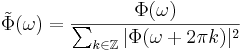$\tilde{\Phi}(\omega) = \frac{\Phi(\omega)}{\sum_{k \in \mathbb{Z}} |\Phi(\omega + 2\pi k)|^2}$

The fourier transform of a B-Spline of order$N$ is (e.g. ):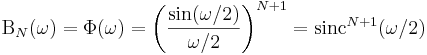$\Beta_N(\omega) = \Phi(\omega) = \left( \frac{\sin(\omega/2)}{\omega/2} \right)^{N+1} = \mathrm{sinc}^{N+1}(\omega/2)$

The following derivation of the sum is borrowed from . For this derivation to work, I set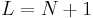$L=N+1$ temporarily: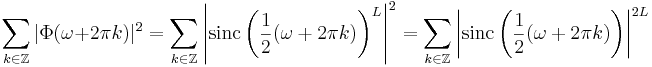$\sum_{k \in \mathbb{Z}} |\Phi(\omega + 2\pi k)|^2 = \sum_{k \in \mathbb{Z}} \left|\mathrm{sinc}\left(\frac{1}{2}(\omega + 2\pi k)\right)^L \right|^2 = \sum_{k \in \mathbb{Z}} \left|\mathrm{sinc}\left(\frac{1}{2}(\omega + 2\pi k)\right) \right|^{2L}$

and because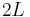$2L$ is always even: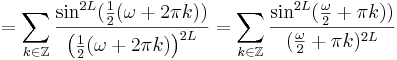$= \sum_{k \in \mathbb{Z}}\frac{\sin^{2L}(\frac{1}{2}(\omega + 2\pi k))}{\left(\frac{1}{2}(\omega + 2\pi k)\right)^{2L}} = \sum_{k \in \mathbb{Z}}\frac{\sin^{2L}(\frac{\omega}{2} + \pi k))}{(\frac{\omega}{2} + \pi k)^{2L}}$

Because of the periodicity it is known that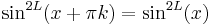$\sin^{2L}(x + \pi k) = \sin^{2L}(x)$

such that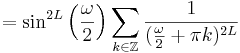$= \sin^{2L}\left(\frac{\omega}{2}\right) \sum_{k \in \mathbb{Z}}\frac{1}{(\frac{\omega}{2} + \pi k)^{2L}}$

And finally the following relation is used: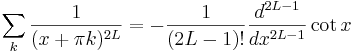$\sum_k \frac{1}{(x + \pi k)^{2L}} = -\frac{1}{(2L-1)!} \frac{d^{2L-1}}{dx^{2L-1}} \cot{x}$

in order to finally obtain: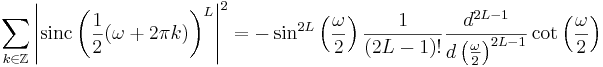$\sum_{k \in \mathbb{Z}} \left|\mathrm{sinc}\left(\frac{1}{2}(\omega + 2\pi k)\right)^L \right|^2 = -\sin^{2L}\left(\frac{\omega}{2}\right) \frac{1}{(2L-1)!} \frac{d^{2L-1}}{d\left(\frac{\omega}{2}\right)^{2L-1}} \cot{\left(\frac{\omega}{2}\right)}$

and with$L = N+1$: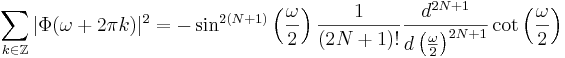$\sum_{k \in \mathbb{Z}} |\Phi(\omega + 2\pi k)|^2 = -\sin^{2(N+1)}\left(\frac{\omega}{2}\right) \frac{1}{(2N+1)!} \frac{d^{2N+1}}{d\left(\frac{\omega}{2}\right)^{2N+1}} \cot{\left(\frac{\omega}{2}\right)}$

Therefore, together with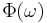$\Phi(\omega)$ this yields: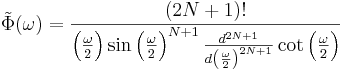$\tilde{\Phi}(\omega) = \frac{(2N+1)!}{\left(\frac{\omega}{2}\right) \sin\left(\frac{\omega}{2}\right)^{N+1} \frac{d^{2N+1}}{d\left(\frac{\omega}{2}\right)^{2N+1}} \cot{\left(\frac{\omega}{2}\right)}}$

and finally substituting for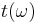$t(\omega)$: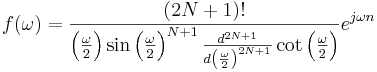$f(\omega) = \frac{(2N+1)!}{\left(\frac{\omega}{2}\right) \sin\left(\frac{\omega}{2}\right)^{N+1} \frac{d^{2N+1}}{d\left(\frac{\omega}{2}\right)^{2N+1}} \cot{\left(\frac{\omega}{2}\right)}} e^{j \omega n}$

As this function is not well defined it is better to use the limit: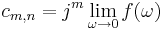$c_{m,n} = j^m \lim_{\omega \rightarrow 0} f(\omega)$

# Examples for a cubic spline

For a cubic spline (N=3) the coefficients are: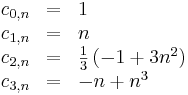$\begin{array}{lcl} c_{0,n} & = & 1 \\ c_{1,n} & = & n \\ c_{2,n} & = & \frac{1}{3}\left( -1 + 3n^2 \right) \\ c_{3,n} & = & -n + n^3 \end{array}$